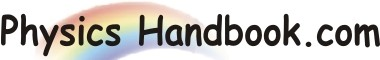HOME TOPICS DEFINITIONS TABLES LAWS INVENTIONS EXPERIMENTS QUIZ VIDEOS
 A B C D E F G H I J K L M N O P Q R S T U V W X Y Z
Superposition Principle
If we are given two charges, the electrostatic force between them can be found by using coulomb's law. However, if a number of charges are present, then force on any charge due to the other charges can be found by superposition principle stated below :
When a number of charges are present, the total force on a given charge is equal to the vector sum of forces due to the remaining other charges on the given charge.
This simply means that we first find the force on the given charge due to the each of the other charges in turn. We then determine the total or net force on the given charge by finding the vector sum of all the forces.
The superposition principle holds good for electric forces and electric fields. This fact has made the mathematical description of electrostatic phenomena simpler that it otherwise would be.
We can use superposition principle to find : net force, net field, net flux, net potential and net potential energy due to a number of charges.